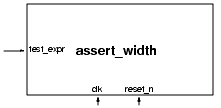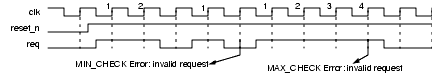assert_width

Ensures that when value of a specified expression is TRUE, it remains TRUE for a minimum number of clock cycles and transitions from TRUE no later than a maximum number of clock cycles.Parameters: severity_level min_cks max_cks property_type msg coverage_level Class: n-cycle assertion

Syntax

assert_width
[#(severity_level, min_cks, max_cks, property_type, msg, coverage_level )]
instance_name (clk, reset_n, test_expr );

Parameters

 severity_level Severity of the failure. Default: ‘OVL_ERROR. min_cks Minimum number of clock edges test_expr must remain TRUE once it is sampled TRUE. The special case where min_cks is 0 turns off minimum checking (i.e., test_expr can transition from TRUE in the next clock cycle). Default: 1 (i.e., same as 0). max_cks Maximum number of clock edges test_expr can remain TRUE once it is sampled TRUE. The special case where max_cks is 0 turns off maximum checking (i.e., test_expr can remain TRUE for any number of cycles). Default: 1 (i.e., test_expr must transition from TRUE in the next clock cycle). property_type Property type. Default: ‘OVL_ASSERT. msg Error message printed when assertion fails. Default: “VIOLATION”. coverage_level Coverage level. Default: ‘OVL_COVER_ALL.

Ports

 clk Clock event for the assertion. The checker samples on the rising edge of the clock. test_expr Expression that should evaluate to TRUE for at least min_cks cycles and at most max_cks cycles after it is sampled TRUE.

Description

The assert_width assertion checker checks the single-bit expression test_expr at each rising edge of clk. If the value of test_expr is TRUE, the checker performs the following steps:

1. Unless it is disabled by setting min_cks to 0, a minimum check is initiated. The check evaluates test_expr at each subsequent rising edge of clk. If its value is not TRUE, the minimum check fails. Otherwise, after min_cks -1 cycles transpire, the minimum check terminates.

2. Unless it is disabled by setting max_cks to 0, a maximum check is initiated. The check evaluates test_expr at each subsequent rising edge of clk. If its value does not transition from TRUE by the time max_cks cycles transpire (from the start of checking), the maximum check fails.

3. The checker returns to checking test_expr in the next cycle. In particular if test_expr is TRUE, a new set of checks is initiated.

Assertion Checks

 MIN_CHECK The value of test_expr was held TRUE for less than min_cks cycles. MAX_CHECK The value of test_expr was held TRUE for more than max_cks cycles. min_cks > max_cks The min_cks parameter is greater than the max_cks parameter (and max_cks >0). Unless the violation is fatal, either the minimum or maximum check will fail.

Cover Points

 test_expr_asserts A check was initiated (i.e., test_expr was sampled TRUE). test_expr_asserted_for_min_cks The expression test_expr was held TRUE for exactly min_cks cycles (min_cks > 0). test_expr_asserted_for_max_cks The expression test_expr was held TRUE for exactly max_cks cycles (max_cks > 0).

assert_change, assert_time, assert_unchange

Example

 assert_width #( ‘OVL_ERROR, 2, 3, ‘OVL_ASSERT, “Error: invalid request”, ‘OVL_COVER_ALL) // severity_level // min_cks // max_cks // property_type // msg // coverage_level valid_request ( clk, reset_n, req == 1); // clock // reset // test_expr

Ensures req asserts for 2 or 3 cycles.